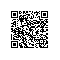# 由RGB到HSV颜色空间的理解

1. RGB模型

2. HSV模型

3. 如何理解RGB与HSV的联系

4. HSV在图像处理中的应用

5. opencv中RGB-->HSV实现

1. RGB模型。PS: 光学的分析

2. HSV模型H是色彩

S是深浅， S = 0时，只有灰度

V是明暗，表示色彩的明亮程度，但与光强无直接联系，（意思是有一点点联系吧）。3. RGB与HSV的联系

v = max(r, g, b)"　　HSV对用户来说是一种直观的颜色模型。我们可以从一种纯色彩开始，即指定色彩角H，并让V=S=1，然后我们可以通过向其中加入黑色和白色来得到我们需要的颜色。增加黑色可以减小V而S不变，同样增加白色可以减小S而V不变。例如，要得到深蓝色，V=0.4 S=1 H=240度。要得到淡蓝色，V=1 S=0.4 H=240度。" --百度百科

4. HSV在图像处理应用

HSV在用于指定颜色分割时，有比较大的作用。

H和S分量代表了色彩信息。

用H和S分量来表示颜色距离，颜色距离指代表两种颜色之间的数值差异
Androutsos等人通过实验对HSV颜色空间进行了大致划分，亮度大于75%并且饱和度大于20%为亮彩色区域，亮度小于25%为黑色区域，亮度大于75%并且饱和度小于20%为白色区域，其他为彩色区域。

对于不同的彩色区域，混合H与S变量，划定阈值，即可进行简单的分割。

HSV的去阴影算法：

Improving shadow suppression in moving object detection with HSV color information

5. RGB --> HSV中的opencv实现

1. struct RGB2HSV_f
2. {
3.     typedef float channel_type;
4.
5.     RGB2HSV_f(int _srccn, int _blueIdx, float _hrange)
6.     : srccn(_srccn), blueIdx(_blueIdx), hrange(_hrange) {}
7.
8.     void operator()(const float* src, float* dst, int n) const
9.     {
10.         int i, bidx = blueIdx, scn = srccn;
11.         float hscale = hrange*(1.f/360.f);
12.         n *= 3;
13.
14.         for( i = 0; i < n; i += 3, src += scn )
15.         {
16.             float b = src[bidx], g = src, r = src[bidx^2];
17.             float h, s, v;
18.
19.             float vmin, diff;
20.
21.             v = vmin = r;
22.             if( v < g ) v = g;
23.             if( v < b ) v = b;       // v = max(b, g, r)
24.             if( vmin > g ) vmin = g;
25.             if( vmin > b ) vmin = b;
26.
27.             diff = v - vmin;
28.             s = diff/(float)(fabs(v) + FLT_EPSILON);  // s = 1 - min/max
29.             diff = (float)(60./(diff + FLT_EPSILON));
30.             if( v == r )
31.                 h = (g - b)*diff;
32.             else if( v == g )
33.                 h = (b - r)*diff + 120.f;
34.             else
35.                 h = (r - g)*diff + 240.f;
36.
37.             if( h < 0 ) h += 360.f;  // h 求值
38.
39.             dst[i] = h*hscale;
40.             dst[i+1] = s;
41.             dst[i+2] = v;
42.         }
43.     }
44.
45.     int srccn, blueIdx;
46.     float hrange;
47. };

RGB --> GRAY的实现 算法：

1. template<typename _Tp> struct RGB2Gray
2. {
3.     typedef _Tp channel_type;
4.
5.     RGB2Gray(int _srccn, int blueIdx, const float* _coeffs) : srccn(_srccn)
6.     {
7.         static const float coeffs0[] = { 0.299f, 0.587f, 0.114f };  // 三分量系数不同，人眼对绿色最敏感，所以G分量系数较大
8.         memcpy( coeffs, _coeffs ? _coeffs : coeffs0, 3*sizeof(coeffs) );
9.         if(blueIdx == 0)
10.             std::swap(coeffs, coeffs);
11.     }
12.
13.     void operator()(const _Tp* src, _Tp* dst, int n) const  // 运算
14.     {
15.         int scn = srccn;
16.         float cb = coeffs, cg = coeffs, cr = coeffs;
17.         for(int i = 0; i < n; i++, src += scn)
18.             dst[i] = saturate_cast<_Tp>(src*cb + src*cg + src*cr);  // 结果
19.     }
20.     int srccn;
21.     float coeffs;
22. };使用钉钉扫一扫加入圈子
+ 订阅